Physical Chemistry : Electromagnetic Spectrum and Radiation

Example Questions

Example Question #1 : Nuclear, Quantum, And Molecular Chemistry

The wavelength of a particular color of red-orange light is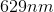.

What is the frequency of this color?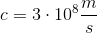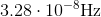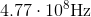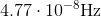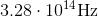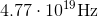Explanation: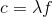, whereis the speed of light,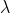is the wavelength, andis frequency.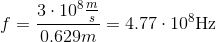Example Question #66 : Physical Chemistry

Microwave radiation falls in the wavelength region of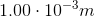.

What is the frequency of microwave radiation that has a wavelength of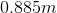?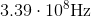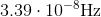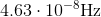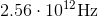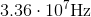Explanation:, whereis the speed of light,is the wavelength, andis frequency.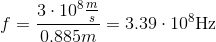Example Question #1 : Electromagnetic Spectrum And Radiation

A researcher analyzes two types of electromagnetic waves. He observes that wave A has a higher amplitude than wave B. What can you conclude about these two waves?

Wave B has the higher wavelength

Wave A has the higher speed

Wave A has the higher wavelength

The relative wavelengths and speed cannot be determined without knowledge of the medium through which the wave is traveling.

The relative wavelengths and speed cannot be determined without knowledge of the medium through which the wave is traveling.

Explanation:

Amplitude of a wave is defined as the maximum peak of oscillation or vibration. Recall that frequency, wavelength, and speed are not dependent on the amplitude and are calculated using the wave equation: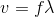Here,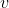is the speed of the wave (which is the speed of light for electromagnetic waves),is the frequency, andis the wavelength. Amplitude of a wave is not present in this equation and, therefore, cannot be used to determine relative values of frequency, wavelength, and speed.

Example Question #68 : Physical Chemistry

If a star approaches earth the electromagnetic waves from the star are perceived to have shorter wavelength whereas if a star moves away from the earth the waves have longer wavelength.

When observing through a telescope, a scientist notices that star A appears red whereas star B appears blue. Which of the following is true regarding these two stars?

Star B is approaching earth

The number of photons from both stars is approximately the same

More than one of these are true

Star A is approaching earth

Star B is approaching earth

Explanation:

The phenomenon described in this question is called redshift and blueshift. All stars emit electromagnetic waves. The perceived wavelength of these waves depends on the relative motion of the object. If an object is approaching the observer (in this case, the earth) then the apparent wavelength of emitted waves decreases whereas if the object moves away then the apparent wavelength increases. This is called the Doppler effect.

To answer this question, we need to know the relative wavelengths of blue and red light. Blue light has wavelength between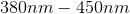whereas red light has wavelength between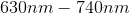. The question states that star A appears red and star B appears blue. Since red has the higher wavelength, star A is going away from the earth. Similarly, since blue has a lower wavelength, star B is approaching earth.

Recall that lower wavelength means higher frequency; therefore, blue has the higher frequency.

Example Question #69 : Physical Chemistry

Microwave rays have a __________ wavelength and a __________ energy than ultraviolet rays.

lower . . . higher

higher . . . higher

lower . . . lower

higher . . . lower

higher . . . lower

Explanation:

To solve this question, we need to first recall the electromagnetic spectrum. Microwaves have very large wavelengths. They are found between visible light and radio waves. Ultraviolet, on the other hand, is found below visible light; therefore, ultraviolet rays have smaller wavelength than microwaves.

To solve for energy we need to use the following equation.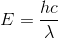Here,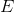is the energy,is Planck’s constant,is speed of light, andis wavelength. Since energy is inversely proportional to wavelength, higher wavelengths will lead to lower energy. This means that microwaves will have lower energy than ultraviolet rays.

Gamma rays have the lowest wavelength and highest energy whereas radio waves typically have the highest wavelength and lowest energy.

Example Question #71 : Physical Chemistry

Two light sources are placed equidistant from a detector. The light from light source A is detected quicker than light from light source B. What can be concluded from these results?

Source A could produce red light whereas source B could produce blue light

The number of photons per unit time is higher from source A

Source A has a higher wavelength

The results are invalid

The results are invalid

Explanation:

The question states that there are two light sources and that both are placed equidistant from a detector. This means that any differences in detection speed is not related to the distance. The question also states that the detector picks up light from source A quicker that light from source B. This means that the speed of the light from source A is faster. Recall that light (or electromagnetic rays) can have varying wavelengths and frequencies; however, the speed of all electromagnetic rays is the same. It doesn’t matter if it is gamma rays or visible light, the speed of light in air is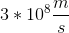. The information given in this question contradicts this; therefore, these results are invalid.

We cannot determine the color of the light for the light sources because we don't have any information regarding their respective wavelength or frequency. The number of photons per unit time refers to the amplitude of the wave, which also cannot be determined from the given information.

Example Question #1 : Electromagnetic Spectrum And Radiation

What is the frequency of microwave radiation that has a wavelength of 0.885m?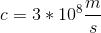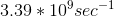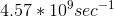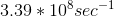None of these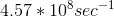Explanation:

To solve this problem, we use the following equation: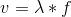Whereis the wavelength andis the frequency.

Wavelength is given, and as we are dealing with the electromagnetic spectrum, we know thatis equal to the speed of light, which is given. We need to solve for frequency, so we arrange the equation as follows: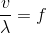Plug in known values and solve.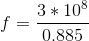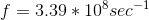Example Question #2 : Electromagnetic Spectrum And Radiation

The wavelength of a particular color of orange light is 629nm.

What is the frequency of this color?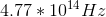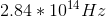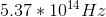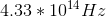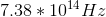Explanation:

To solve this problem, we use the following equation:Whereis the wavelength andis the frequency.

Wavelength is given, and as we are dealing with the electromagnetic spectrum, we know thatis equal to the speed of light, which is given. We need to solve for frequency, so we arrange the equation as follows:Plug in known values and solve.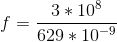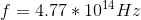Example Question #3 : Electromagnetic Spectrum And Radiation

A local FM radio station broadcasts at a frequency of 99.0MHz.

What is the wavelength at which the station is broadcasting?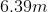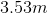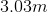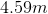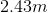Explanation:

To solve this problem, we use the following equation:Whereis the wavelength andis the frequency.

Rearrange the equation to solve for wavelength.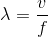Plug in known values and solve.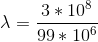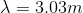All Physical Chemistry Resources